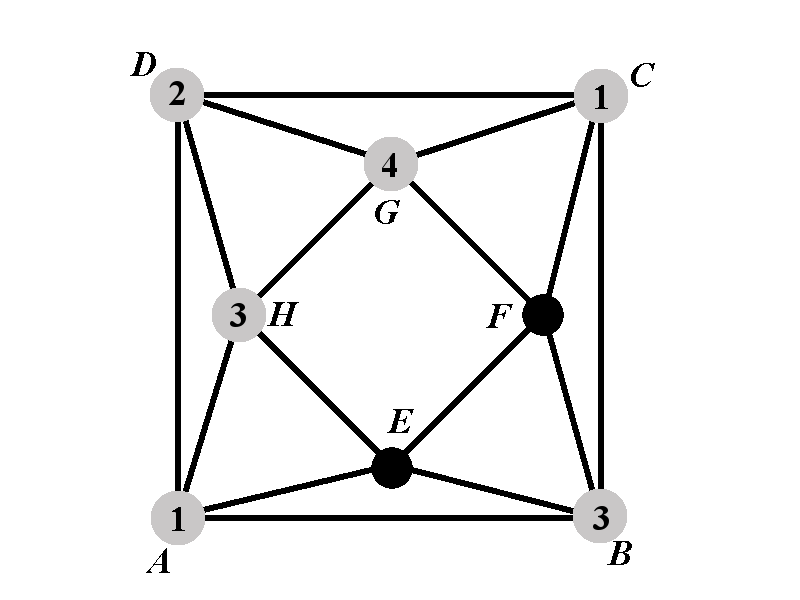Back to: Chromatic Polynomial
##### Case 1.1: Calculation of $$P_{1.1^{\circ}}(k)$$Observe that the vertex $$F$$ cannot have any of the colors $$1$$, $$3$$, $$4$$, while the vertex $$E$$ cannot have any of the colors $$1$$ and $$3$$.

There are $$k-3$$ colors available for the vertex $$F$$. For each of the choices, there are $$k-3$$ color available for the vertex $$E$$ because the vertex $$E$$ cannot have the colors $$1$$, $$3$$, and the color that is assigned to the vertex $$F$$.

Hence we obtain $P_{1.1^{\circ}}(k)=(k-3)^2.$